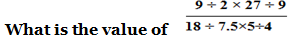# RRB JE Practice Questions | Mathematics – Day-03

Dear Aspirants,

Mathematics is a complex web. So only most of the applicant’s thought is RRB Mathematics questions are tough to work out. It’s really not, to score the maximum mark in this section you need of regular practice on RRB JE Maths every day.

Start to practice Quiz for RRB Mathematics section and reveal the answers with detailed explanation. We have taken much care on the explanation part that to understand the RRB Mathematics questions easily. Take RRB Mathematics quiz regularly to perform better on  RRB JE Maths section without any hurdle.

[WpProQuiz 5275]

1)  What is the value of 26 – [25 – {? – (22 – 21.5)}] = 3

a) 2.5

b) 35

c) 3

d) 3.5

2) What is the value of 3 ÷ [(18 – 15) ÷ {(14 -12) ÷ (2 + 8/13)}] =?

a) 17/13

b) 13/17

c) 68/13

d) 68/17

3)  What is the value of 16 ÷ 8 (13 -12) × 4 +13-17 = ?

a) 8

b) 16

c) 4

d) 12

4)  What is the value of  ⅛ ÷ ⅛ of  ⅛  = ?

a) 8

b) ⅛

c) 1/64

d) 64

5) What is the value of (16 ÷ 4) ÷ 2 +(36 ÷  18) × 2 + (10 ÷ 5) × (3 ÷ 2) = ?

a) 18

b) 16

c) 3

d) 9

6) What is the value of 1255.5 ÷ (18 × 15.5) × 2 = ?

a) 4.5

b) 9

c) 18

d) 36

7) What is the value of (3535 ×  0.35 ÷ 5) × 6 – 3.7 = ?

a) 1481.3

b) 1484.7

c) 1481

d) 1484.3

8)a) 4.5

b) 5.5

c) 4.8

d) 18

9) What is the value of   of 92% of 24/23 of 650 = 85 + ?

a) 18

b) 21

c) 19

d) 28

10)  What is the value of of 2/7 of ? = 426

a) 2490

b) 2565

c) 2475

d) 2485

26 – [25 – {? – 0.5}] = 3

26 – [25 – ? + 0.5] = 3

0.5 +? = 3

x = 2.5

3 ÷ [ 3 ÷ {2 ÷  34/13}] =?

3 ÷ [3 ÷ { 2×13 /34} =?

3 ÷ [(3 ×34)/26]=?

(3×26)/(3×34)=13/17

16 ÷ 8 × 4 – 4 = ?

2 × 4 – 4=8-4 = 4

⅛  ÷ 1/64 =?

⅛  × 64 = 8

4÷2+2×2+2 × 3/2 = ?

2 + 4+ 3 =9

1255.5 ÷ 279 × 2 =?

4.5 × 2=9

(3535 × 0.07) × 6 -3.7 =?

247.45 × 6 – 3.7=?

1484.7 – 3.7 = 1481

13.5 / (2.4 × 1.25) =13.5 / 3= 4.5

4×2×13= 85+?

104 -85 = 19